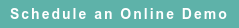# RoyaltyStat Blog

### Posts by Topic

Naive analysts use the normal (Gauss-Laplace) distribution to compute the mean and standard deviation, or quartiles, without verifying if the data are abiding. Double fault is committed when they propose a “broad and unconvincing” range of data, such as the interquartile range (IQR), which may become useless to determine arm’s length (or reasonable) royalty rates. In practice, the interquartile range of royalty rates is used to help settle licensing disputes in tax or intellectual property valuation.

To better understand royalty rates in a specific industry, such as in chemicals, pharmaceuticals, software or medical devices, we consider a large sample of independent license agreements, subject to the following search restrictions (data were retrieved from RoyaltyStat on September 20, 2016):

a) Industry: Medical Devices;

b) Agreement Type (Including): Patent;

c) Royalty Base: Net Sales (= Revenue, Turnover);

d) Related Parties: Exclude.

These large industry-wide samples may be used as a “sanity check” to test the reasonableness of the proposed range of royalty rates based on usually a small count of “comparables”. Using standard practice, the summary statistics of the 1,155 unique (non-duplicate) medical device agreements include the mean = 6.6%, standard deviation = 6.5%, and the quartiles are Q1 = 3%, the median (Q2) = 6.6%, and Q3 = 8%. The coefficient of variation is practically one, suggesting that the data have long tails. It is prudent to further explore the royalty rates distribution and examine the probable long tails to see if the selected data depart from normality.

First, using John Tukey's filter, we find that high outliers, measured by Q3 + 1.5 IQR, where the interquartile range is IQR = (Q3 – Q1), consist of medical device agreements containing royalty rates ≥ 15.5%. See J. Tukey, Exploratory Data Analysis, Addison-Wesley, 1977, p. 44. Observations beyond this outer fence are outliers, and they can be used as “comparables” only under special circumstances. Outliers distort the computation of the mean and standard deviation, and make them unreliable. Prima facie, the Medtronic proposed range of royalty rates (based on seven judgments or non-random “comparables”) between 0.5% and 20% include outliers when we consider this repository of available medical device license agreements. The existence of outliers suggests that in the Medtronic tax controversy a “test of reasonableness” (aka “sanity check”) was disregarded by the company expert. As a result, it's not surprising that the U.S. Tax Court (TC) rejected the expert report because it contained a “broad and unconvincing” range of royalty rates. See Medtronic v Commissioner of Internal Revenue, TC Memo 2016-112, Docket 6944-11, filed June 9, 2016, located online at: https://www.ustaxcourt.gov/UstcInOp/OpinionViewer.aspx?ID=10819

Second, we can use the probability plot as a visual test of the data normality assumption. We can also use the Anderson-Darling (AD) test, but the visual probability plot is likely to be more persuasive. The normal distribution is symmetrical around the mean. The empirical distribution of royalty rates is asymmetric, with long tails skewed to the right. As we show below, based on this large industry sample, the distribution of medical devices royalty rates departs from the normal distribution. The top probability plot shows that the data deviate from the theoretical diagonal lines. However, we get a good fit using the lognormal distribution, again as we can see on the bottom plot below. As a result of the lack of normality, the standard formulae used to compute summary statistics are inappropriate, and those statistics are misleading.

We should respect the actual (instead of an assumed) data distribution and use the appropriate formulae to compute the mean and standard deviation.  E.g., we know that the mean of the normal distribution is μ and the standard deviation is σ; however, these parameters are different from the lognormal distribution in which the mean is α = exp (μ + 0.5 σ2) and the standard deviation is β = SQRT[exp (2 μ + σ2)  × (exp 2) – 1], where μ is the location and σ is the scale parameter. See J. Aitchison & J. Brown, The Lognormal Distribution, Cambridge University Press, 1969, p. 8. According to C. Kleiber & S. Kotz, Statistical Size Distributions in Economics, John Wiley & Sons, 2003, p.108, "the pioneering study marking initial use of the lognormal distribution was [Robert] Gibrat's thesis of 1931," which was published in Paris as Les Inégalités Économiques, Librairie de Recueil Sirey. Wikipedia contains a detailed description of the parameters of the lognormal distribution and the Anderson-Darling (AD) test for normality. Also see: http://www.itl.nist.gov/div898/handbook/eda/section3/eda3669.htm

In conclusion, it's important to know the comparable data used to determine an arm's length range of royalty rates, and to test the reasonableness of the results using a larger or parent data set. Assuming that the selected comparables produce royalty rates (or profit margins) that obey the normal distribution may be perilous, particularly when we know that economic variables with positive values such as royalty rates tend to follow the lognormal distribution. In this regard, it's important to understand that standard statistical formulae to compute the mean, standard deviation, and quartiles belong to the normal distribution, and such formulae become different when the underlying data distribution depart from the ubiquitous normal assumption. RoyaltyStat has a built-in statistical tool to generate histograms and probability plots (based on royalty rates from your selected comparables), including ML estimates of the mean, standard deviation, and quartiles of the normal and lognormal distributions.Published on Sep 27, 2016 11:42:30 AM

Ednaldo Silva (Ph.D.) is founder and managing director of RoyaltyStat. He helped draft the US transfer pricing regulations and developed the comparable profits method called TNNM by the OECD. He can be contacted at: esilva@royaltystat.com

RoyaltyStat provides premier online databases of royalty rates extracted from unredacted license agreements
and normalized company financials (income statement, balance sheet, cash flow). We provide high-quality data, built-in analytical tools, customer training and attentive technical support.Topics: Royalty Rates